# Calculating Volatility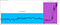USDTRY pair showing a low and high volatility environments. (Image by Author)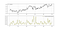EURUSD in the first panel with the 10-period Standard Deviation in the second panel. (Image by Author)
`def volatility(Data, lookback, what, where):      for i in range(len(Data)):    try:      Data[i, where] = (Data[i - lookback + 1:i + 1, what].std())        except IndexError:            pass            return Data`

# Creating the Relative Volatility Index

`my_ohlc_array = volatility(my_ohlc_array, 14, 3, 4)# We are saying that we want to apply the volatility function seen above onto our OHLC array using a lookback period of 14 and applying the calculation on the closing price (hence, the fourth column which is indexed at 3). Then, we want to output the result in the fifth empty column which is indexed at 4`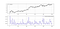EURUSD in the first panel with the 14-period Standard Deviation in the second panel. (Image by Author)
`for i in range(len(my_ohlc_array)):           if my_ohlc_array[i, std_col] > my_ohlc_array[i - 1, std_col]:                    my_ohlc_array[i, positive_ch] = my_ohlc_array[i, std_col]   else:        my_ohlc_array[i, positive_ch] = 0            for i in range(len(my_ohlc_array)):           if my_ohlc_array[i, std_col] < my_ohlc_array[i - 1, std_col]:                    my_ohlc_array[i, negative_ch] = my_ohlc_array[i, std_col]  else:        my_ohlc_array[i, negative_ch] = 0# The variable std_col refers to the column where the Standard Deviation is stored# The variable positive_ch refers to the Positive Change column that will be populated# The variable negative_ch refers to the Negative Change column that will be populated`
`def ma(Data, lookback, what, where):        for i in range(len(Data)):      try:        Data[i, where] = (Data[i - lookback + 1:i + 1, what].mean())                    except IndexError:                pass    return Datamy_ohlc_array = ma(my_ohlc_array, lookback, positive_ch, up_ma)my_ohlc_array= ma(my_ohlc_array, lookback, negative_ch, down_ma)# The variable up_ma refers to where we want to store the averaged values of the positive change while the down_ma refers to where we want to store the averaged values of the negative change`
`def relative_volatility_index(Data, lookback, what, where):Data = volatility(Data, lookback, what, where)        for i in range(len(Data)):                if Data[i, where] > Data[i - 1, where]:                        Data[i, where + 1] = Data[i, where]        else:            Data[i, where + 1] = 0                for i in range(len(Data)):                if Data[i, where] < Data[i - 1, where]:                        Data[i, where + 2] = Data[i, where]        else:            Data[i, where + 2] = 0                            Data = ma(Data, lookback, where + 1, where + 3)    Data = ma(Data, lookback, where + 2, where + 4)# RVI    for i in range(len(Data)):              Data[i, where + 5] = 100 * Data[i, where + 3] / (Data[i, where + 3] + Data[i, where + 4])        return Data`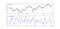GBPUSD hourly data in the first panel with the 14-period RVI in the second panel. (Image by Author)

# Back-testing a Contrarian Strategy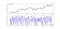AUDUSD hourly data in the first panel with the 3-period RVI in the second panel. (Image by Author)

# Risk Management Snapshot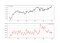EURUSD hourly data with the 50-period Exponential Average True Range. (Image by Author).

Written by